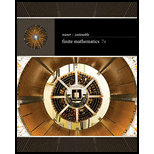# In Exercises 85–88, use the given logical equivalence to rewrite the given sentence. [ HINT: See Example 5(c).] It is not true that either I am Julius Caesar or you are a fool. DeMorgan’s law.### Finite Mathematics

7th Edition
Stefan Waner + 1 other
Publisher: Cengage Learning
ISBN: 9781337280426### Finite Mathematics

7th Edition
Stefan Waner + 1 other
Publisher: Cengage Learning
ISBN: 9781337280426
Chapter A, Problem 86E
Textbook Problem
33 views

## In Exercises 85–88, use the given logical equivalence to rewrite the given sentence. [HINT: See Example 5(c).]It is not true that either I am Julius Caesar or you are a fool. DeMorgan’s law.

To determine

The representation of the sentence “It is not true that either I am Julius Caesar or you are a fool.” Using De Morgan's law of logical equivalence.

### Explanation of Solution

De Morgan's law states that If there are two statements p and q, then

(pq)(p)(q)(pq)(p)(q)

Consider the statement, “it is not true that either I am Julius Caesar or you are a fool”.

Here, p: I am Julius Caesar,

q: you are a fool.

Evaluate the condition, either I am Julius Caesar or you are a fool by applying disjunction.

pq

Then evaluate the it is not true that either I am Julius Caesar or you are a fool, using negation of pq

### Still sussing out bartleby?

Check out a sample textbook solution.

See a sample solution

#### The Solution to Your Study Problems

Bartleby provides explanations to thousands of textbook problems written by our experts, many with advanced degrees!

Get Started

Find more solutions based on key concepts
In Exercises 4756, solve the given equation for the indicated variable. 5x+1=1125

Finite Mathematics and Applied Calculus (MindTap Course List)

Perform each operation and simplify: a. (x2+1)(12x1/2)x1/2(2x)(x2+1)2 b. 3xx+2+3x+2

Applied Calculus for the Managerial, Life, and Social Sciences: A Brief Approach

Elementary Technical Mathematics

Evaluate the integrals in Problems 7-18. 10.

Mathematical Applications for the Management, Life, and Social Sciences

Finding a Limit What is the limit of g(x)=x as x approaches ?

Calculus: Early Transcendental Functions (MindTap Course List)

True or False: x2+1x3dx may be solved using a trigonometric substitution.

Study Guide for Stewart's Single Variable Calculus: Early Transcendentals, 8th